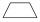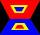# Isosceles trapezoid v3

In an isosceles trapezoid ABCD is the size of the angle β = 81°
Determine size of angles α, γ and δ.

Result

α =  81 °
γ =  99 °
δ =  99 °

#### Solution:Leave us a comment of example and its solution (i.e. if it is still somewhat unclear...):Be the first to comment!## Next similar examples:

1. Isosceles trapeziumTrapezoid YSED (YS||ED) is isosceles. The size of the angle at vertex Y is 17 degrees. Calculate the size of the angle at vertex E.
2. Rectangular trapezoidHow many inner right angles has a rectangular trapezoid?
3. Trapezium 2Trapezium has an area of 24 square cms. How many different trapeziums can be formed ?
4. Obtuse angleWhich obtuse angle is creating clocks at 17:00?
5. Degrees to radiansConvert magnitude of the angle α = 9°39'15" to radians:
6. TVsProduction of television sets increased from 3,500 units to 4,200 units. Calculate the percentage of production increase.
7. Conference148 is the total number of employees. The conference was attended by 22 employees. How much is it in percent?
8. Percents - easyHow many percent is 432 out of 434?
9. Simplify 2Simplify expression: 5ab-7+3ba-9
10. Apples 2James has 13 apples. He has 30 percent more apples than Sam. How many apples has Sam?
11. GlovesI have a box with two hundred pieces of gloves in total, split into ten parcels of twenty pieces, and I sell three parcels. What percent of the total amount I sold?
12. Unknown numberIdentify unknown number which 1/5 is 40 greater than one tenth of that number.
13. InequationSolve the inequation: 5k - (7k - 1)≤ 2/5 . (5-k)-2
14. New refrigeratorNew refrigerator sells for 1024 USD, Monday will be 25% discount. How much USD will save, and what will be the price?
15. ClassIn a class are 32 pupils. Of these are 8 boys. What percentage of girls are in the class?
16. EquationSolve the equation: 1/2-2/8 = 1/10; Write the result as a decimal number.
17. NumberWhat number is 20 % smaller than the number 198?